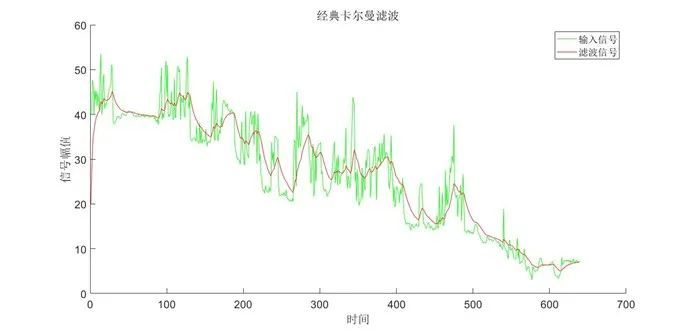# 滤波算法：经典卡尔曼滤波

2023-02-14 来源：互联网 字号：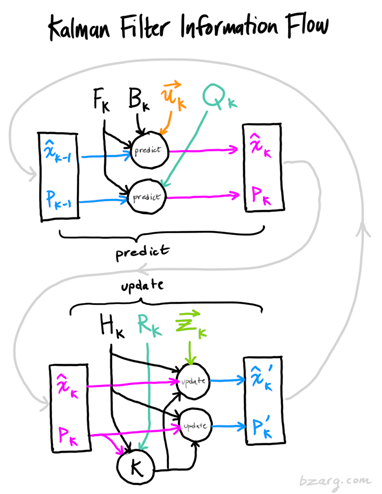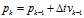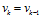​如果我们加入额外的控制量，比如加速度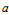，此时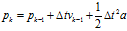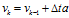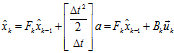(3)

​同时，我们认为我们对系统的估计值并非完全准确，比如运动物体会突然打滑之类的，即存在一个协方差为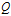的噪声干扰。因此，我们需要对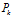​加上系统噪声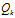来保证描述的完备性。综上，预测步骤的表达如下所示：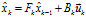(4)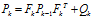(5)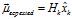(6)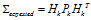(7)

​我们假设观测值为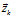。同时由于观测数据同样会存在噪声干扰问题，比如传感器噪声等，我们将这种噪声的分布用协方差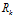表示。此时，观测值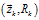与估计值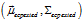处于相同的状态空间，但具有不同的概率分布，如图2所示：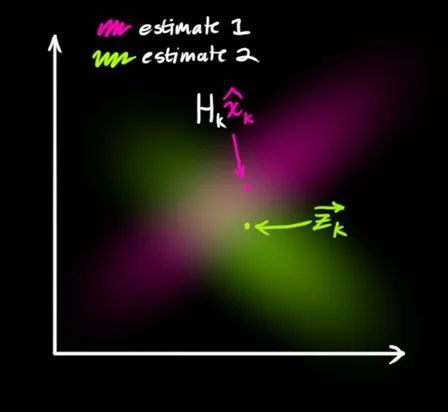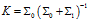(8)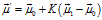(9)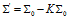(10)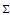描述高斯分布的协方差，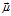表示高斯分布的均值，矩阵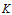称为卡尔曼增益矩阵。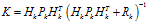(11)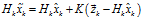(12)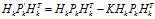(13)

​式中，称为卡尔曼增益。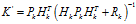(14)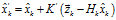(15)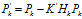(16)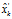即为我们所得到的最优估计值，同时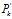为其对应的协方差矩阵。在实际应用中，只需要使用式(4)、式(5)以及式(14)至式(16)这5个方程即可实现完整的卡尔曼滤波过程。

2. 算法实现

function output = kalmanFilter(data, Q, R, N)

if ~exist('Q', 'var')

Q = 0.01;

end

if ~exist('R', 'var')

R = 1;

end

if ~exist('N', 'var')

N = 0;

end

X = 0;

P = 1;

A = 1;

H = 1;

output = zeros(size(data));

for ii = N + 1 : length(data)

X_k = A * X;

P_k = A * P * A' + Q;

Kg = P_k * H' / (H * P_k * H' + R);

z_k = data(ii - round(rand() * N));

X = X_k + Kg * (z_k - H * X_k);

P = (1 - Kg*H) * P_k;

output(ii) = X;

end

end

3. 算法分析